# Free Numerical Aptitude Tests, Free Numerical Reasoning Tests and Free Numeracy Tests with Detailed Answer Explanations

## What Is a Numerical Reasoning Test?

Numerical reasoning tests are used to measure your ability to work with and understand numbers, as part of your decision making.

Numerical reasoning test questions are an essential component of cognitive, aptitude and psychometric tests.

Numerical reasoning test questions typically include a wide range of numerical test question styles, such as; tables & graphs, ratios, percentages and number series. The style of numerical test questions and the level of difficulty is customised to the job you are applying for.

Numerical reasoning test questions offer you a large amount of information to process in a very short time, making these types of questions fairly challenging.

### Please note: Numerical test questions are customised to the job you are applying for. For example, if you apply for a job with one of the banks you will get a different style of numerical test questions than when you  apply for a job with federal police.

Our expert psychometricians have developed a list of successful preparation packages to ensure that you pass your numerical reasoning test with flying colours:

1. Real practice numerical tests, customised to your job, with detailed answer explanations.
2. Online courses.
3. Face-to-face or Skype video tutoring with our expert psychometricians.

# Numerical Question Styles: Get to know the question style that you will get

Our expert psychometricians have collected information about the 6 styles of numerical reasoning test question that are most commonly used.

It is important to get to know the different styles of numerical reasoning test question so you will be adequately prepared for your numerical reasoning test.

Free Numerical Reasoning Practice Tests

Below you will be able to practice short numerical reasoning tests. Each practice test will focus on different styles of numerical test question.

We also offer a detailed answer explanation at the end of each practice test. We would strongly advise that you read the detailed answer explanations to learn how to answer similar numerical test questions in your real test.

Free numerical reasoning test 1

This practice numerical reasoning test has several numerical test question styles that measure your numerical reasoning skills.

This free numerical test includes:

12 test questions

You have 9 minutes to complete this test

Free Numerical Reasoning Test 2

This second practice numerical reasoning test has a different set of numerical test question styles that also measure your numerical reasoning skills.

This free numerical test includes:

8 test questions

You have 4 minutes to complete this test

Free Numerical Reasoning Test 3

This third practice numerical reasoning test has a different style of numerical test question which include a timer per test question.

This free numerical test includes:

8 test questions

You have 6 minutes to complete this test

# Job Customised Numerical Reasoning Tests - Practice Questions That You'll See on Your Real Test

While most candidates will receive an invite to take a numerical reasoning test, the style of test questions is likely to be very different. The style of numerical test questions is customised to the job you are applying for.

You must practice for the correct style of numerical test questions to avoid wasting your time.

Our expert psychometricians have collected example numerical reasoning test questions with step-by-step explanations:

We have collected some example numerical reasoning test questions of different styles to assist you in understanding what type of questions you are likely to be asked to solve in your real numerical test.

Getting to know the type of numerical test questions and the correct techniques required to solve them is very important to allow you to successfully pass your numerical reasoning test.

Each example numerical reasoning test also has a detailed step-by-step answer explanation.

### “Tables & Graphs” numerical test questions

The aim of numerical reasoning tests is to measure your ability to understand information presented in a numerical form. One of the most popular forms of presenting numerical data is tables and graphs.

In a graph and table style numerical test question, you will be asked to quickly read and interpret information presented in a table or a graph. Your task will be to find the relevant information, then use this information to find the correct answer.

### What to expect in a table and graph test question?

• A typical numerical test question in this style will have a table with numerical information presented in many columns and lines or a graph, such as a line or bar graph.
• The table or graph will typically have far more information than you need to answer the question.
• Your task will be to identify the relevant information and use it to answer the test question.

Example numerical reasoning test question - table

Winter clothing company sales: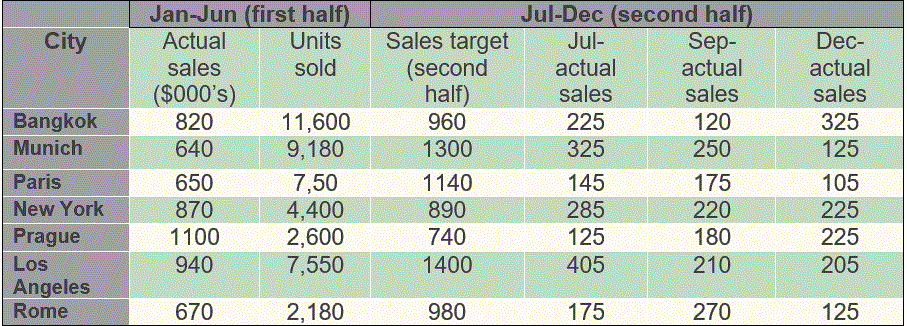What is the approximate ratio between differences in first half actual sales and second half sales target in Munich and Rome?

2:1

5:3

2:3

3:5

1:2

Munich’s second half target was 1300, actual sales in first half were 640, and difference is 1300-640=660. Rome’s second half target was 980, actual sales in first half were 670, and difference is 980-670=310. So the ratio between differences is 660/310= approx. 2:1

Example numerical reasoning test question – graph

Price fluctuations from January to June for three varieties of flowers: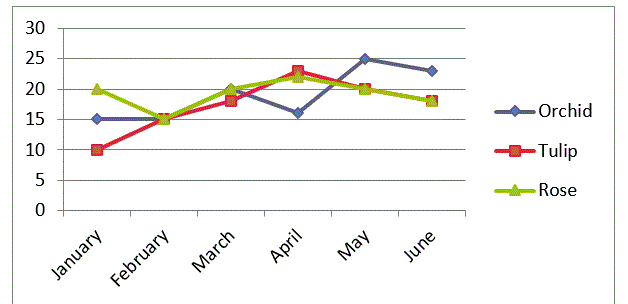Which of the following options represents the biggest price increase in a single month?

Tulip from January to February

Tulip from March to April

Orchid from April to May

Rose from February to March

Based on the graph, the price of a Tulip (the red line) increased by \$5 from January to February, and \$5 from March to April. An Orchid’s price (the blue line) increased from April to May by nearly \$10, while the price of a Rose (the green line) increased by \$5 from February to March. Therefore, the Orchid recorded the biggest increase.

Getting the right techniques to solve table and graph style numerical test questions, in the quickest way possible, is crucial to your success.

Remember: don't waste time practising numerical test question styles that you will not have in your real numerical reasoning test.

### “Ratio” numerical test questions

Ratios seem to be a very popular style to measure candidates’ numerical reasoning skills. A ratio can be displayed as a straightforward question about a ratio of X to Y or it can be incorporated in an indirect way such as in currency conversions. In the indirect ratios you will always be given ratios so you can use them to calculate or convert the currency or any other unit.

Example for a direct ratio numerical test question:

The number of divorces in two cities for June to November: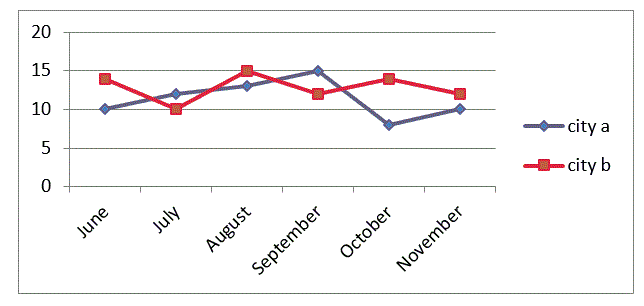For city a, what is the ratio between the difference in divorces from June to July and the difference from September to October?

1:3

2:3

2:7

3:4

According to the graph for city a (the blue line), divorces increased from June to July by 2 and from September to October they deceased by 7; therefore the ratio is 2:7

Remember: don't waste time practising numerical test question styles that you will not have in your real numerical reasoning test.

### “Percentages” numerical test questions

Percentages seem to appear in most, if not all, numerical reasoning tests. Therefore, it is important to ensure that you know how to calculate percentages and what the concept of percentage means.

In a typical percentage numerical test question, you will need to calculate a percentage change (for example the percentage change in sales between two months) or you will be given a percentage increase or decrease of a figure and you will be asked to calculate the final figure (for example a price increase or decrease).

Example of a percentage numerical test question:

Jewellery sales in April and October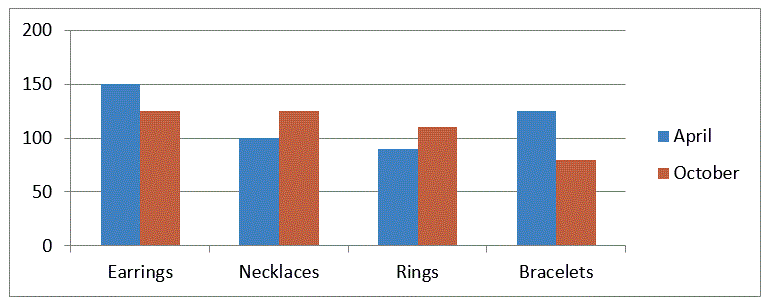Approximately, what percentage of jewellery sales in October were Bracelets?

Around 12%

Around 18%.

Around 25%.

Around 31%

Based on the bar graph, sales of Bracelets are represented by the red bar. As the precise figures are not given for each jewellery item, we need to approximate the number of items sold. To find what is the total number of items sold in October we need to add the approximate number of items sold for each type of jewellery. Based on the bar graph, the approximate total number of items sold in October is: around 125 Earrings + around 125 Necklaces + around 110 Rings + around 80 Bracelets = around 440 items. If the number of bracelets sold in October was around 80 then if we divide 80 by 440 and multiply by 100 to get the percentage, we get a little over 18%. Therefore, the nearest option to this figure is 18%.

### “Number series” numerical test questions

Numerical test questions that include number series can be separated into  two groups. The first group is one long sequence of numbers that make up a number series. The second group is a Sudoku type of number series. Typically appearing in a 3 by 3 table.

In the number series questions, the sequence of numbers have logical rules and patterns that make them a group. The logical rules and patterns have to do with the specific position of each number in the sequence.

Your task in solving number series questions is to find the unique patterns and logical rules for each series, then use these patterns and logical rules to find a missing number.

Learning the specific techniques of how to quickly analyse number series is crucial, as you are given a very short amount of time to complete each test question.

Example of a number series test question – line number series

What is the missing number that should take the place of the question mark?

19, 22, 26, ?, 37, 44, 52

To answer this question you need to identify the pattern in the numbers. The simplest option which we recommend to start with is finding the gaps between the numbers and to check whether they form a pattern. In this case 22-19=3, 26-22=4, 44-37=7, 52-44=8. So, we get the following sequence of numbers: 3, 4, ?, ?, 7, 8. It should be easy to see that this forms a pattern of 3, 4, 5, 6, 7, 8… Therefore, the missing number is 26+5=31.

Example number series test question – Sudoku number series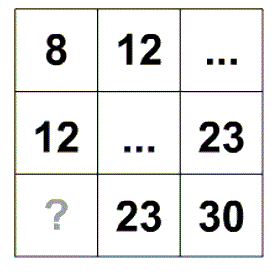To answer this question you need, as in the previous example, to identify that patterns in the numbers. However, in this case you have more than one series of numbers. First thing you need to decide in this type of test question is whether you wish to tackle the numbers horizontally or vertically. If you decide on horizontally, we recommend that you only focus on pairs of numbers – i.e. 8, 12 and 23,30, to identify that pattern. If we use that same method, as in the previous question, we can find that the gaps are 12-8=4 and 30-23=7. Therefore, we can conclude that the pattern for the horizontal number series is +4 to move from the left column to the middle column and +7 to move from the middle column to the column on the right. If we follow this pattern then the missing number is 23-4=19.

Another example of numerical aptitude test question in this category:

This type of numerical test questions include numbers sequences that are displayed in triangles or long grids.To answer this question you need to find the patterns or number sequences in this example. If we examin the numbers in the top line: 4, 12, 36... we can see that each consecutive number is product of multiplying the previous number by 3. For example, 4 x 3 = 12 and 12 x 3 = 36. Based on this the next number will be 36 x 3 = 108. The numbers at the bottom are created by subtracting 3 from the numbers above. For example, 4 - 3 = 1, 12 - 3 = 9, 36 - 3 = 33 and 108 - 3 = 105. The missing number at the bottom will be 108 x 3 = 324 then 324 - 3 = 321. So the missing numbers are 108 and 321.

To ensure you are ready for your numerical test, simply go to our home page and selelct your Classification and Job-level and cick GO to get the following professional preparation packages:

• ### 1-on-1 personal tutoring delivered by experienced psychometric test trainers.Need Help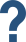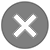Need HelpPlease fill out the form below and one of our Psychometric test experts will contact you soon.
Your message was sent. We will contact you shortly.
There was a problem sending your message. Please try again in a few minutes.